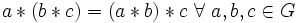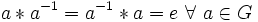# Group

(diff) ← Older revision | Latest revision (diff) | Newer revision → (diff)

## Definition

A group is a set$G$ equipped with three additional operations:

• A binary operation$*$ called multiplication, or product
• A unary operation denoted as$g \mapsto g^{-1}$ called the inverse map
• A constant element denoted$e$

such that the following conditions hold:

•$a * (b * c) = (a * b) * c \ \forall \ a, b, c \in G$
•$a * e = e * a = a \ \forall \ a \in G$
•$a * a^{-1} = a^{-1} * a = e \ \forall \ a \in G$

## Importance

Groups arise in differential geometry, primarily in the following contexts:

• As symmetries, or automorphisms, of geometric structures
• As structure groups of bundles
• As fundamental groups or higher (co)homotopy and (co)homology groups
• As manifolds themselves. Notions of relevance here are topological group and Lie group